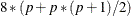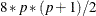# The COUNTREG Procedure

### Computational Resources

The time and memory that PROC COUNTREG requires are proportional to the number of parameters in the model and the number of observations in the data set being analyzed. Less time and memory are required for smaller models and fewer observations. Also affecting these resources are the method that is chosen to calculate the variance-covariance matrix and the optimization method. All optimization methods available through the METHOD= option have similar memory use requirements.

The processing time might differ for each method, depending on the number of iterations and functional calls needed. The data set is read into memory to save processing time. If not enough memory is available to hold the data, the COUNTREG procedure stores the data in a utility file on disk and rereads the data as needed from this file. When this occurs, the execution time of the procedure increases substantially. The gradient and the variance-covariance matrix must be held in memory. If the model has p parameters including the intercept, then at leastbytes are needed. If the quasi-maximum likelihood method is used to estimate the variance-covariance matrix (COVEST=QML), an additionalbytes of memory are needed.

Time is also a function of the number of iterations needed to converge to a solution for the model parameters. The number of iterations that are needed cannot be known in advance. The MAXITER= option can be used to limit the number of iterations that PROC COUNTREG does. The convergence criteria can be altered by nonlinear optimization options available in the PROC COUNTREG statement. For a list of all the nonlinear optimization options, see Chapter 6: Nonlinear Optimization Methods.